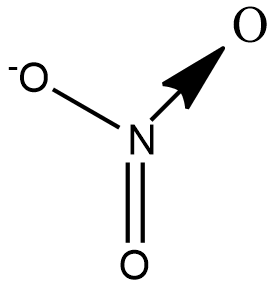In $N{{O}_{3}}^{-}$ ion, number of bond pair and lone pair of electrons on nitrogen are:(A) 2,2(B) 3,1(C) 1,3(D) 4,0Verified
74.6k+ views
Hint: Draw the structure of $N{{O}_{3}}^{-}$ representing all the bonds. Nitrogen cannot have 5 bonds although it shows oxidation state of +5, hence there is a possibility of coordinate bond. The bond pair for every coordinate bond is taken as 1.

Let us draw the structure of $N{{O}_{3}}^{-}$ to understand the types of bond present in the ion:We see that there are 2$\sigma$ bonds, 1$\pi$bond and 1 coordinate bond. Theoretically the coordinate bond is equivalent to a double bond but differs in terms of reactivity towards an incoming ion.
A coordinate bond also called a dative covalent bond is a type of covalent bond in which both electrons come from the same atom. Nitrogen forms a coordinate bond with Oxygen as it cannot have 5 bonds. Although 2 electrons are shared in a coordinate bond, the number of bond pairs is taken to be 1.
Hence the number of bond pairs are 4 and the number of lone pairs is 0.
Therefore, the correct answer is option (D).

Note: Nitrogen shows the oxidation state of +5 in $N{{O}_{3}}^{-}$ ion. However, nitrogen cannot have 5 bonds attached to itself. This is because nitrogen does not have vacant orbitals to expand to its octet. From the subsequent period, the elements have vacant d orbitals and can expand their octet. For e.g. phosphorus can have 5 bonds attached to itself as seen in the molecule $PC{{l}_{5}}$,but $NC{{l}_{5}}$cannot exist.